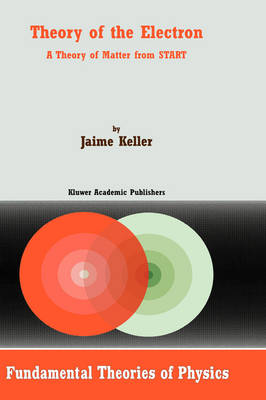•Theory of the Electron: A Theory of Matter from START - Fundamental Theories of Physics v. 115 (Hardback)

(author)
£117.00
Hardback Published: 01/02/2001
• Not available

This product is currently unavailable.

In the first century after its discovery, the electron has come to be a fundamental element in the analysis of physical aspects of nature. This book is devoted to the construction of a deductive theory of the electron, starting from first principles and using a simple mathematical tool, geometric analysis. Its purpose is to present a comprehensive theory of the electron to the point where a connection can be made with the main approaches to the study of the electron in physics. The introduction describes the methodology. Chapter 2 presents the concept of space-time-action relativity theory and in chapter 3 the mathematical structures describing action are analyzed. Chapters 4, 5, and 6 deal with the theory of the electron in a series of aspects where the geometrical analysis is more relevant. Finally in chapter 7 the form of geometrical analysis used in the book is presented to elucidate the broad range of topics which are covered and the range of mathematical structures which are implicitly or explicitly included. The book is directed to two different audiences of graduate students and research scientists: primarily to theoretical physicists in the field of electron physics as well as those in the more general field of quantum mechanics, elementary particle physics, and general relativity; secondly, to mathematicians in the field of geometric analysis.